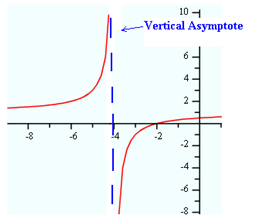Discontinuities

Discontinuities of the graph are the undefined points of the rational function. Remember when simplifying rational expressions sometimes there are excluded values which create points of discontinuity.

Points of discontinuity or exception points can occur in two different forms:

• the denominator of the rational function equals zero and the numerator does not creating a vertical asymptote
• the denominator and numerator of the rational function equal zero creating a hole.

Remember that a vertical asymptote is a vertical line that the graph of the function approaches but never intersects. When the denominator equals zero and the numerator does not, this discontinuity is a vertical asymptote.